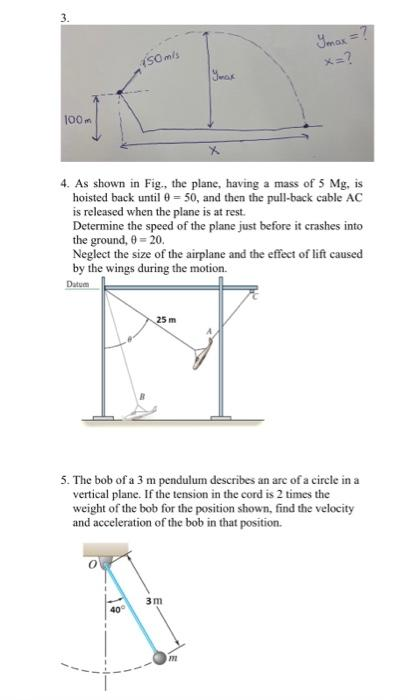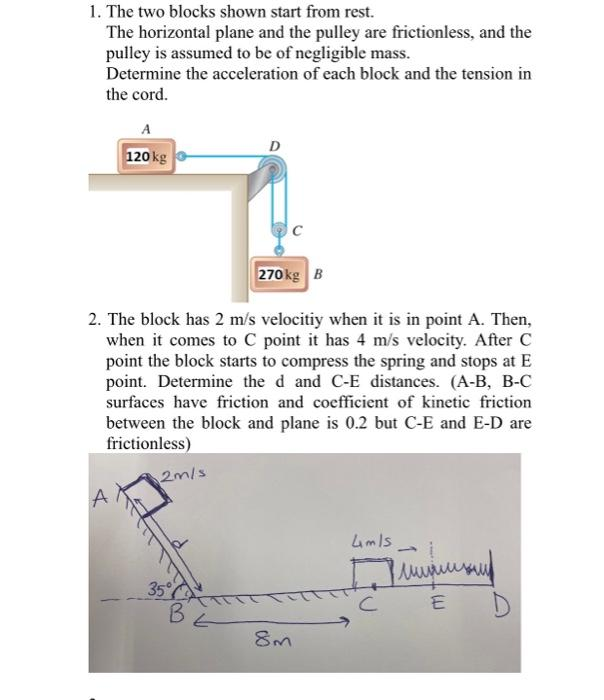Home / Expert Answers / Mechanical Engineering / 4-as-shown-in-fig-the-plane-having-a-mass-of-5mg-is-hoisted-back-until-50-and-then-the-pull-pa788

# (Solved): 4. As shown in Fig, the plane, having a mass of 5Mg, is hoisted back until =50, and then the pull ...4. As shown in Fig, the plane, having a mass of , is hoisted back until , and then the pull-back cable is released when the plane is at rest. Determine the speed of the plane just before it crashes into the ground, . Neglect the size of the airplane and the effect of lift caused by the wings during the motion. 5. The bob of a pendulum describes an are of a circle in a vertical plane. If the tension in the cord is 2 times the weight of the bob for the position shown, find the velocity and acceleration of the bob in that position. 1. The two blocks shown start from rest. The horizontal plane and the pulley are frictionless, and the pulley is assumed to be of negligible mass. Determine the acceleration of each block and the tension in the cord. 2. The block has velocitiy when it is in point A. Then, when it comes to C point it has velocity. After C point the block starts to compress the spring and stops at point. Determine the and distances. (A-B, B-C surfaces have friction and coefficient of kinetic friction between the block and plane is but C-E and E-D are frictionless)

We have an Answer from Expert